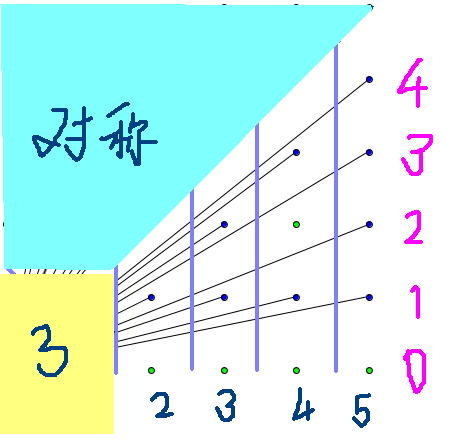# 博客

## #32. 【SDOI2008】仪仗队

2018-03-15 17:35:43 By NaCl

### 题图### 欧拉函数

#### 计算函数表

$a$ 为质数 $\Leftrightarrow$ $\phi(a)==a-1$.

//phi：函数表；p：质数表；m：最后一个被搜的因数
void table(){
phi = 1;
for(int i=2; i<N; i++){
if(!m[i]) //i 为质数，加入质数表
p[pt++]=m[i]=i, phi[i] = i - 1;
for(int j=0; j<pt && p[j] * i < N; j++){
// 遍历所有已查明的质数
m[k]=p[j];
if(m[i]==p[j]){
phi[k]=phi[i]*m[i];
break;
}
else phi[k]=phi[i]*(p[j]-1);
}
}
}

## 评论

poorpool

luv_letters
%%%%%%%%%%%%%
lris

NaCl
@poorpool @Iris 你们忽略了博文的第 2 行（抖机灵）
NaCl
https://www.luogu.org/paste/c925ro4w